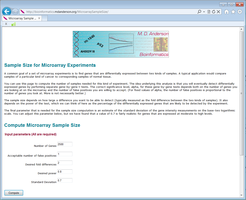### Department of Bioinformatics and Computational Biology

Home > Public Software > Sample Sizes

Sample Sizeshidden row for table layout Overview Description A tool to compute the number of samples needed to detect expression changes for a microarray experiment. Development Information URL https://biostatistics.mdanderson.org/MicroarraySampleSize/ Language HTML, Javascript, MySQL Current version 1.0 Help and Support Contact MDACC-Bioinfo-IT-Admin@mdanderson.org

## Sample Sizes

A common goal of a set of microarray experiments is to find genes that are differentially expressed between two kinds of samples. A typical application would compare samples of a particular kind of cancer to corresponding samples of normal tissue.

You can use this page to compute the number of samples needed for this kind of experiment. The idea underlying this analysis is that you will eventually detect differentially expressed genes by performing separate gene-by-gene t-tests. The correct significance level, alpha, for these gene-by-gene tests depends both on the number of genes you are looking at on the microarray and the number of false positives you are willing to accept. (For fixed values of alpha, the number of false positives is proportional to the number of genes you look at. More is not necessarily better.)

The sample size depends on how large a difference you want to be able to detect (typically measured as the fold difference between the two kinds of samples). It also depends on the power of the test, which we can think of here as the percentage of the differentially expressed genes that are likely to be detected by the experiment.

The final parameter that is needed for the sample size computation is an estimate of the standard deviation of the gene intensity measurements on the base-two logarithmic scale. You can adjust this parameter below, but we have found that a value of 0.7 is fairly realistic for genes that are expressed at moderate to high levels.

### Underlying Model

These sample size computations are based on the assumption that the expression of each gene is normally distributed on the log scale. We assume further that the variance is the same in the two experimental groups, and thus the only difference between the groups is the mean. The acceptable number of false positives is divided by the number of genes to compute a per-gene significance level alpha that would be expected to produce that number of false positives. (As a consequence, asking for 0 false positives will require infinitely many samples.) Finally, we pretend that gene expression measurements are independent, and perform the usual computations to determine the sample size needed for a t-test with an underlying normal distribution.